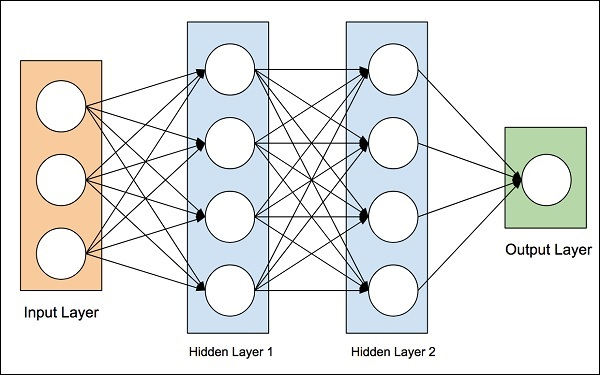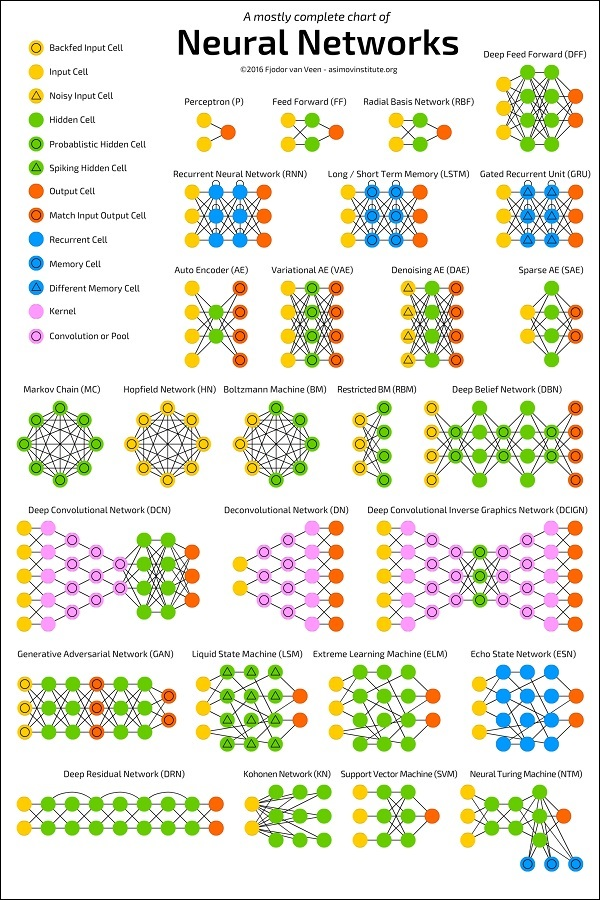# blog posts# Artificial neural networks (ANNs)

An artificial neural network(ANN) is a computing system that has been inspired by the biological neural network and its functions.

An ANN is a model that is based on connections among several nodes which they called artificial nodes. This type of computation (ANN) mimics the neurons and their connection in the biological brain.

Like the biological brain, each connected neuron transmits data and signals to another.

An artificial neuron that gets the transmitted signal, can then process it and again signal another artificial neuron. Generally, this can be considered as a recursive action.

The signals among artificial neurons can be numerical and the output signal of a neuron is the result of non-linear computation and functions of the sum of its input.

The connections between two neurons are called edges. Both neurons and edges have their own weight which will be adjusted as the learning process continues.

Increasing or decreasing weight can be mean as strength or weakness of a signal. Generally, in artificial neural networks neurons gather into the layers, and each layer’s information and signals are different.# Training

How can an artificial neural network learn? the answer is, ANN learns by the processing of input data, in this case, training or examples.

In the neural network, the difference between prediction and target output of data called error. Then the weight of the network will be adjusted through learning rules and errors.

After adjustment of weight, the ANN training process can be terminated and it is ready to perform like a trained model.

As we mentioned in a previous post, in supervised learning, in supervised learning an expert is needed to train models with lots of examples. For example, an expert has to label the input data as for example this picture is a dog, this is a cat, and so on. But in the neural network, there is no need to labeled input data for training the model.

In ANN model learns the rule by extraction and detection of features for example fur, tails, whiskers, and cat-like faces.

# ANN architectures

In the following images, you can see the Artificial Neural Network Architecture and then various architectures of ANN that have been developed so far.# Learning techniques in artificial neural network

In the following list, you can see the list of techniques that have been used in the artificial neural network.

• Supervised Learning: is a learning approach that trains a model with several examples that have been labeled by an expert
• Unsupervised Learning: type of learning that learns the structural similarity from the nature of the data
• Reinforcement Learning: reinforcement learning is one of the most vital types of learning which learns the value of each permitted action in a specific state of the environment.
• Offline Learning: This type of learning which is also called Bach Learning, adjusts the weight of neurons and their connection only after training the training data.
• Online Learning: In online learning, the system uses and trains from every single sample. It means that the model adjusts neuron and connector’s weights after presenting each training sample.

# Some application of artificial neural networks

• Handwriting Recognition
• Traveling Salesman Problem
• Image Compression
• Stock Exchange Prediction